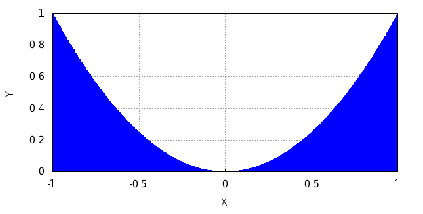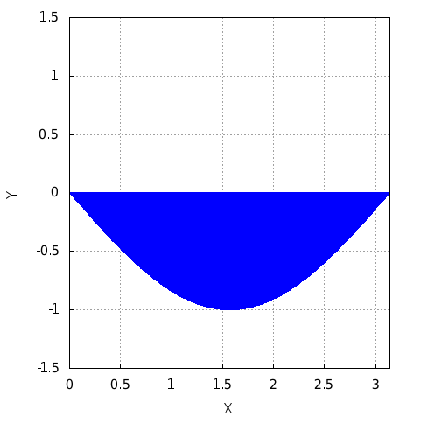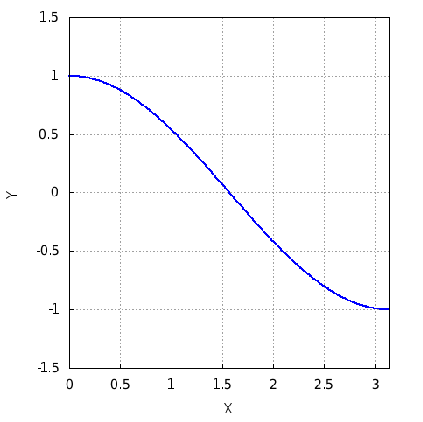## Basic Integral Calculus

January 4, 2019
The purpose of this worksheet is to illustrate, how a person who knows Calculus 2 can simply compute, the area under a parabola, in his head, assuming that the parabola has a simpliﬁed set of coeﬃcients, as well as the simpliﬁed domain from -1 to +1. The plot below shows the simple case in which:

$y={x}^{2},x\in \left[-1,+1\right]$Later, this worksheet will provide a similar clue, about the integration of a trig function. However, an in-depth explanation of that sort of integration is beyond the scope of this worksheet.

The function of the parabola has both an indeﬁnite, and a deﬁnite integral. The indeﬁnite integral is deﬁned like so:

$\int {x}^{2}dx=\frac{1}{3}{x}^{3}+C$

Which means that it would be valid for any range, if not for the fact that it must have the arbitrary constant ’C’, to allow for an unknown amount of area that might have preceded any vaule of ’x’ which we fed in. To avoid this confusion, Cal 2 teaches its Students the deﬁnite integral ﬁrst.

But the deﬁnite integral can trivially be derived from the indeﬁnite integral. To write it, this is what we put:

${\int }_{x=-1}^{+1}{x}^{2}dx={\left[\frac{1}{3}{x}^{3}\right]}_{-1}^{+1}$

This can be taken to say, ’Compute the indeﬁnite integral for x=+1 (the end of the interval), then the indeﬁnite integral for x=-1 (the beginning of the interval), and subtract the value that results from the beginning of the interval, from the value that results from the end of the interval; the arbitrary constant will then cancel out.’ And so, when plugging in +1 and then -1, into the cubic equation that is the integral of the quadratic either way, in a practiced fashion, this becomes:

$\left[\frac{1}{3}{\left(+1\right)}^{3}-\frac{1}{3}{\left(-1\right)}^{3}\right]=\frac{2}{3}$

And so the area under this parabola has been computed analytically, which means that an exact, algebraic solution was found, which is by no means an approximation.

If we wanted to compute the deﬁnite integral:

${\int }_{x=-1}^{+1}xdx$

It would be easy to prove, that the answer is zero...

${\left[\frac{1}{2}{x}^{2}\right]}_{-u}^{+u}$

The reason why, is the simple fact that for any Real Number ’u’:

${\left(-u\right)}^{2}={\left(+u\right)}^{2}$

Therefore,

$a{\left(+u\right)}^{2}-a{\left(-u\right)}^{2}=0$

Similarly, the only information we might have about a trig function to integrate, could be:

$y=-sin\left(x\right),x\in \left[0,\pi \right]$Thus, the deﬁnite integral can in fact be wrtitten:

${\int }_{x=0}^{\pi }\phantom{\rule{0em}{0ex}}\left(-sin\left(x\right)\right)dx$

The interval to compute would become:

${\left[+cos\left(x\right)\right]}_{0}^{\pi }$

This can be seen as a confusing example, simply because an easy mistake for Humans to make, is in the use of the negative signs. This is what cos(x) looks like over the same domain:In reality, the interval would need to be computed:

$cos\left(\pi \right)-cos\left(0\right)=\left(-1\right)-\left(+1\right)=-2$

Computer Algebra Systems (’CAS’) will tend to get such use of the negative signs right, as long as the problem was keyed in correctly. However, the best ways in which Humans can recognize mistakes, such as simply to observe that the trig function’s plot extends below the x-axis, and should therefore have negative area ’under’ the curve, is unavailable to a modern CAS, or to an AI for that matter, such that a Human using a CAS can provide enhanced insurance against mistakes.

The way Human Mathematicians usually write the above integral is instead to put the negative sign in front, like this:

$-{\int }_{x=0}^{\pi }sin\left(x\right)dx$

Without any real controversy, this yields the same result, like so:

$-{\left[-cos\left(x\right)\right]}_{0}^{\pi }=-\left(+2\right)=-2$

Dirk Mittler